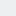# Tag: kp hart

## HAPPY PI DAY with Mathematician KP Hart

Pi – Questions and answers about that wonder number, 3.14 from Dutch Mathematician, K.P. Hart!

## Pi – Who Comes Up With the Numbers After 3.14?

Here’s Mathematician KP Hart’s Math Question and Answer for today! Read it, sear it onto your...

## YOUR MONDAY MATH with Mathematician KP Hart: Prime Number Systems

Here’s Mathematician KP Hart’s Math Question and Answer for Monday, March 28th! *********** You...

## YOUR MONDAY MATH with Mathematician KP Hart: Grasping Infinity

How is it that humans cannot grasp the concept of infinity? Infinity is a notion that can make you...

## YOUR FRIDAY MATH with Mathematician KP Hart: A Zero for Languages

Does there exist something for languages that is comparable to the number zero for mathematics and...

## YOUR MONDAY MATH with Mathematician KP Hart: DOES 0.9999 = 1?

Here’s Mathematician KP Hart’s Math Question and Answer for Monday, March 7th! *********** Is...

## YOUR FRIDAY MATH with Mathematician KP Hart – Original Music

Here’s Mathematician KP Hart’s Math Question and Answer for Friday, March 4th! Can original music...

## YOUR MONDAY MATH with Mathematician KP Hart – Logics

Here’s Mathematician KP Hart’s Math Question and Answer for Monday, February 22nd! Logics Is there...

## YOUR MONDAY MATH with Mathematician KP Hart – What Does the Infinite Look Like?

Here’s Mathematician KP Hart’s Math Question and Answer for Monday, February 1st! Why can’t I...

## YOUR FRIDAY MATH with Mathematician KP Hart – The Shape of the Earth

In honor of the rapper B.o.B’s hilarious assertion that the Earth is flat and also in honor...